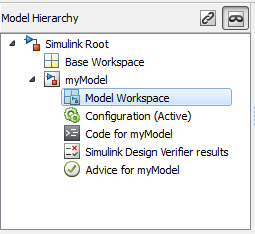## Change Model Workspace Data

When you use a model workspace to contain the variables that a model uses, you choose a source to store the variables, such as the model file or an external MAT-file. To modify the variables at the source, you use a different procedure depending on the type of source that you selected.

### Change Workspace Data Whose Source Is the Model File

If the data source of a model workspace is the model file, you can use Model Explorer or MATLAB® commands to modify the stored variables (see Use MATLAB Commands to Change Workspace Data).

For example, to create a variable in a model workspace:

1. Open the Model Explorer. On the Modeling tab, click Model Data Editor or press Ctrl+H.

2. In the Model Explorer Model Hierarchy pane, expand the node for your model, and select the model workspace.3. Select Add > MATLAB Variable.

You can similarly use the Add menu or toolbar to add a `Simulink.Parameter` object to a model workspace.

To change the value of a model workspace variable:

1. Open the Model Explorer. On the Modeling tab, click Model Explorer.

2. In the Model Explorer Model Hierarchy pane, select the model workspace.

3. In the Contents pane, select the variable.

4. In the Contents pane or in Dialog pane, edit the value displayed.

To delete a model workspace variable:

1. Open the Model Explorer. On the Modeling tab, click Model Explorer.

2. In the Model Explorer Model Hierarchy pane, select the model workspace.

3. In Contents pane, select the variable.

4. Select Edit > Delete.

### Change Workspace Data Whose Source Is a MAT-File or MATLAB File

You can use Model Explorer or MATLAB commands to modify workspace data whose source is a MAT-file or MATLAB file.

To make the changes permanent, in the Model Workspace dialog box, use the button to save the changes to the MAT-file or MATLAB file.

1. Open the Model Explorer. On the Modeling tab, click Model Explorer.

2. In the Model Explorer Model Hierarchy pane, right-click the workspace.

3. Select the Properties menu item.

4. In the Model Workspace dialog box, use the button to save the changes to the MAT-file or MATLAB file.

To discard changes to the workspace, in the Model Workspace dialog box, use the button.

### Changing Workspace Data Whose Source Is MATLAB Code

The safest way to change data whose source is MATLAB code is to edit and reload the source. Edit the MATLAB code and then in the Model Workspace dialog box, use button to clear the workspace and re-execute the code.

To save and reload alternative versions of the workspace that result from editing the MATLAB code source or the workspace variables themselves, see Export Workspace Variables and Importing Workspace Variables.

### Use MATLAB Commands to Change Workspace Data

To use MATLAB commands to change data in a model workspace, first get the workspace for the currently selected model:

```hws = get_param(bdroot, 'modelworkspace'); ```

This command returns a handle to a `Simulink.ModelWorkspace` object whose properties specify the source of the data used to initialize the model workspace. Edit the properties to change the data source.

Use the workspace methods to:

• List, set, and clear variables

• Evaluate expressions in the workspace

• Save and reload the workspace

For example, the following MATLAB code creates variables specifying model parameters in the model workspace, saves the parameters, modifies one of them, and then reloads the workspace to restore it to its previous state.

```hws = get_param(bdroot, 'modelworkspace'); hws.DataSource = 'MAT-File'; hws.FileName = 'params'; hws.assignin('pitch', -10); hws.assignin('roll', 30); hws.assignin('yaw', -2); hws.saveToSource; hws.assignin('roll', 35); hws.reload; ```

To programmatically access variables for the purpose of sweeping block parameter values, consider using `Simulink.SimulationInput` objects instead of modifying the variables through the programmatic interface of the model workspace. See Optimize, Estimate, and Sweep Block Parameter Values.